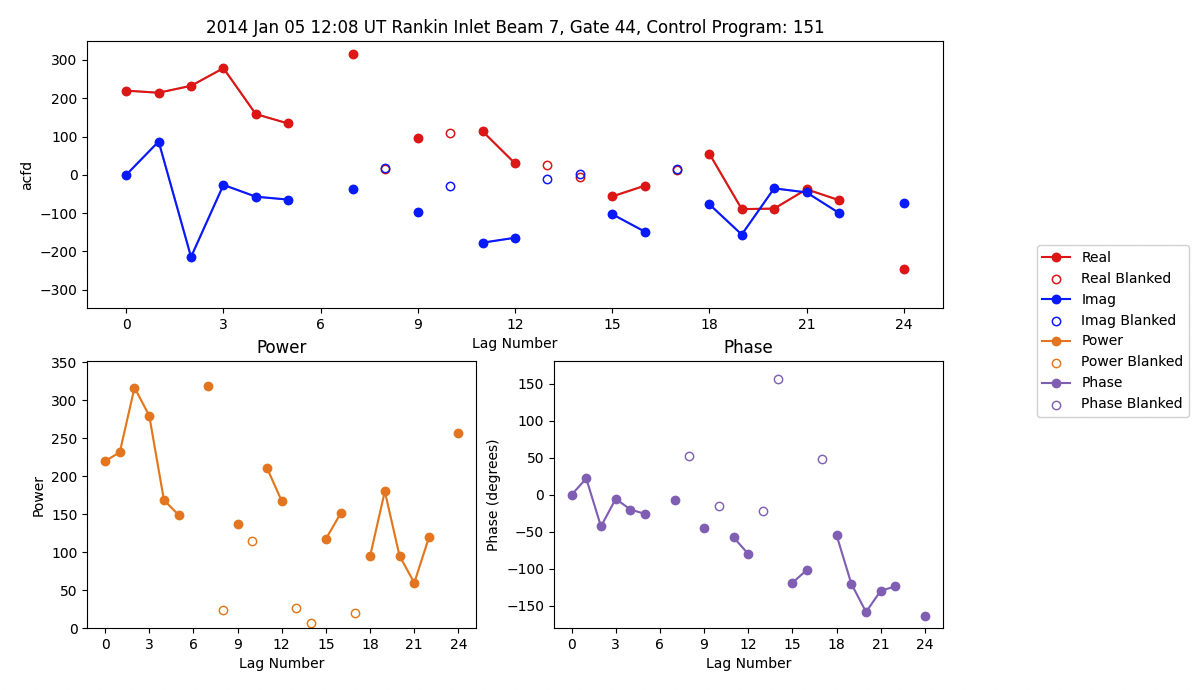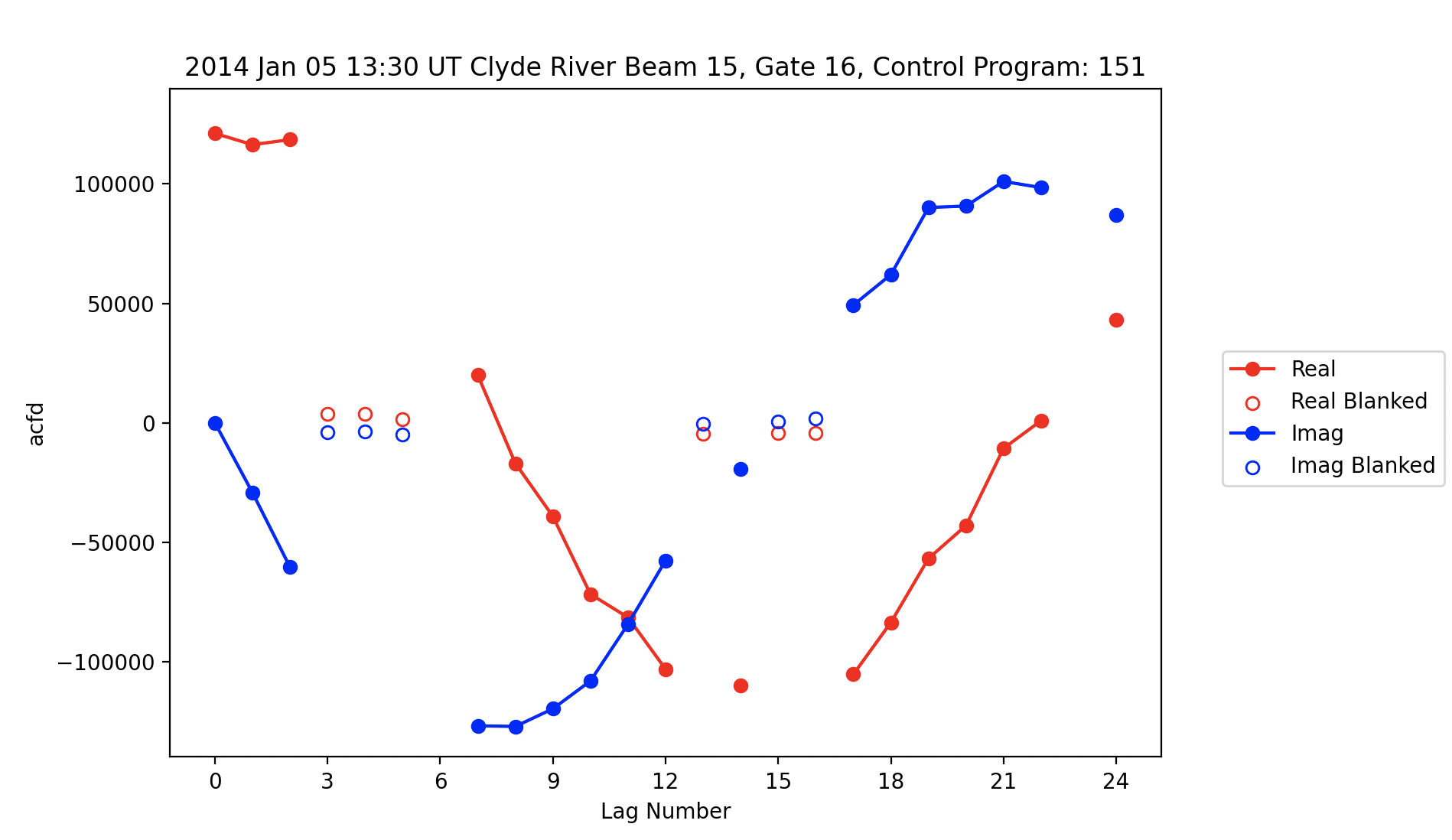### Auto-Correlation Function Plots

`plot_acfs` simply plots the Auto-Correlation Function (ACF) of the imaginary and real parts in the selected RAWACF file.

Basic code to plot ACFs from a RAWACF file would look like:

``````import matplotlib.pyplot as plt
import pydarn

file = "20180101.0000.01.rkn.rawacf"

pydarn.ACF.plot_acfs(rawacf_data)
plt.show()
``````Parameter Action
beam_num=0 beam number to plot
gate_num=0 gate number to plot
parameter='acfd' parameter to pick between acfd or xcfd plotting
scan_num=0 the scan number to plot
start_time=None plot the closest beam scan to the given start time (overrides the scan number if set)
ax matplotlib axes object
normalized=True normalizes the parameter data with the associated power 0 value
real_color='red' Real part of the parameter line color
imaginary_color='blue' Imaginary part of the parameter line color
plot_blank=False Determine if blanked lags should be plotted
blank_marker='o' Choice of marker to indicate blanked lags are a dot (general python markers accepted)
legend=True plot a legend
kwargs arguments passed in matplotlib line_plot for real and imaginary plots

If blank lags are present in the data, it will look similar to the following:

``````import pydarn
import matplotlib.pyplot as plt
from datetime import datetime

rawacf_file = '20140105.1200.03.cly.rawacf'